Journal of Water Resources and Ocean Science
Volume 5, Issue 6, December 2016, Pages: 93-103

Vertical Water Velocity Distribution Using Fine Different Sediment Concentrations in Circular Open Channel

Mohamed Gamal Mohamed Abdalla

Irrigation and Hydraulics Dept., Faculty of Engineering, Mansoura University, Mansoura, Egypt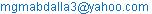Mohamed Gamal Mohamed Abdalla. Vertical Water Velocity Distribution Using Fine Different Sediment Concentrations in Circular Open Channel. Journal of Water Resources and Ocean Science. Vol. 5, No. 6, 2016, pp. 93-103. doi: 10.11648/j.wros.20160506.13

Received: October 22, 2016; Accepted: November 1, 2016; Published: December 16, 2016

Keywords: Bed Load, Suspended Load, Circular Channel, ADV Instrument, Velocity Distribution

Contents

1. Introduction

Moving sediments in several parts of flow is considered the main item for used formulas that calculate sediment transport rate. These formulas are often separated into bed load, suspended, and wash load.

Bed load moves in the lower part of the channel or river in form of rolling, sliding with bed or in saltating over the bed.

Suspended load usually found in the lower to middle parts of the flow, and moves with the flow velocity in the stream.

The oldest known sediment transport study was done around 4000 years ago in China .

A significant work has been done in the last century in the field of sediment transport. All studies could be classified into physical studies and mathematical studies.

Physical studies are done by doing experiments in laboratory flumes or by taking field observations.

Laboratory studies are not well representative of the river system as it is difficult to represent a river by a laboratory flume. So a lot of assumptions are usually incorporated in laboratory studies. Still these laboratory studies are important for verification of other studies and also to understand basic concepts of river flow and sediment transport. One of the oldest and still widely used studies was done by Newton . Also, Suryanarayana and Shen  did extensive laboratory flume studies for three different sediment particle sizes using uniform sediment grain size for each experimental run.

The vertical distribution of water velocity with suspended sediment concentration has been investigated in turbulent flow by many researchers.

The problems of sediment-laden flows are important to river engineers and geomorphologists. This problem related to the field such as coastal sediment transport and transport of solids in pipelines.

Studying the vertical distribution of sediment concentration with water velocity is based on the logarithmic velocity distribution given by Prandtl mixing-length hypothesis and on von-Karman similarity hypothesis (Schlichting and Gersten, ).

The vertical water velocity profile is described by a ‘log-law’ in case of flow over smooth bed in open channels without moving sediment. Through the measurements in the whole turbulent boundary layer zone, the ‘log-law’ could be only applied to the near wall region with the von Karman constant κ equals 0.4 and the integrating constant could be 5.29.

Coles  developed a semi-empirical equation that modifies the ‘log-law’ based on wake-strength function. This developed equation could be applied to the whole turbulent boundary layer zone.

Several researchers Coles , Nezu and Rodi  and Nezu and Nakagawa  used ‘wake-function’ to account the deviation from the "log-law" and they recommended that the measured water velocity cannot be fitted only by log-law.

Vanoni  and Einstein and Chien  found that a smaller value of "k" than the universal one (k=0.4) gave an excellent results with their experimental results of velocity and suspension distributions except the zone near bottom. They concluded that, the von-Karman coefficient κ reduced with the increase in suspension concentration.

New models for velocity distribution were made by Itakura and Kishi  and Coleman  to concentration of vertical sediments using constant value of "k".

Thacker and Lavelle  explained the effects of hindered settling on the concentrations of vertical sediment profiles. Also, Woo et al.  estimated the vertical concentration of particles "sand particles" using the log law and power law based on the hindered settling effects.

Mazumder and Ghoshal  proposed a new theoretical model for vertical sediment and velocity concentration profiles using fine, medium and coarse sediment.

Pellachini  provided a new relationship for the bed load transport of the sand fraction when gravel particles remain at rest.

Milad et al.  provided a study to set up a physical model based on the key variables in the previous transport equation to study the transport process in shallow grade culverts and storm sewers.

The mechanics of sediment transport is important for civil and hydraulics engineering structures such as culverts, dams, and around bridge piers as the resulted erosion of the bed. This erosion can damage the environment and expose or unsettle the foundations of the structure. (Grant et al., ). So, in this paper, studying the effect of different fine sediment concentrations on the vertical velocity profiles in circular pipe was experimentally studied using "ADV" (Acoustic Doppler Velocimeter) instrument. Also, the bed load was separated from the total load by a trap and the effect of time on the settling process was studied. New relationships between vertical velocity and suspended load concentration were also deduced.

2. Experimental Work

All tests were exerted in a circular tilting flume of 20 cm diameter, and 5.0 m long, figure 1, in irrigation and hydraulics laboratory in Mansoura University.

Figures (2, 3 and 4) show the flume, plan and its side view and input tank with a specified water volume of 67.5 cm × 154 cm × 25 cm to give constant water volume of 0.26 m3. This input tank used for mixing all used sediment concentrations.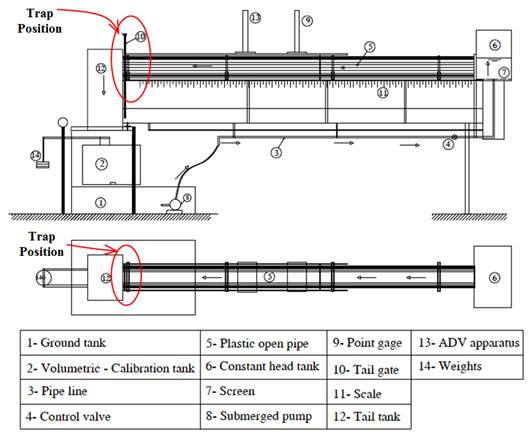Figure 1. Elevation and plan of the used flume.Figure 2. Flume "plan".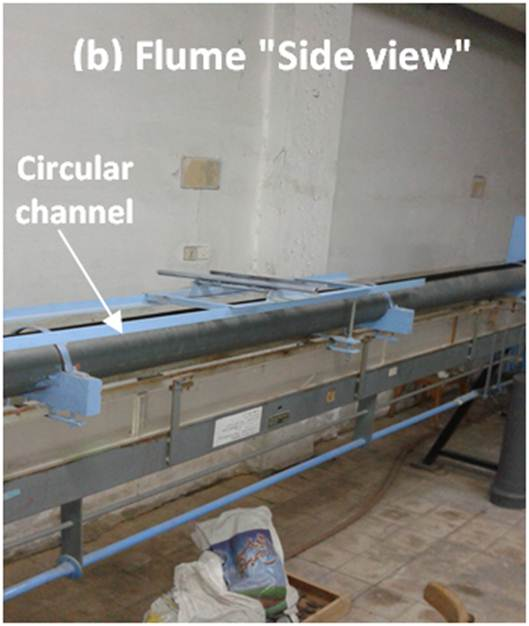Figure 3. Flume "side view".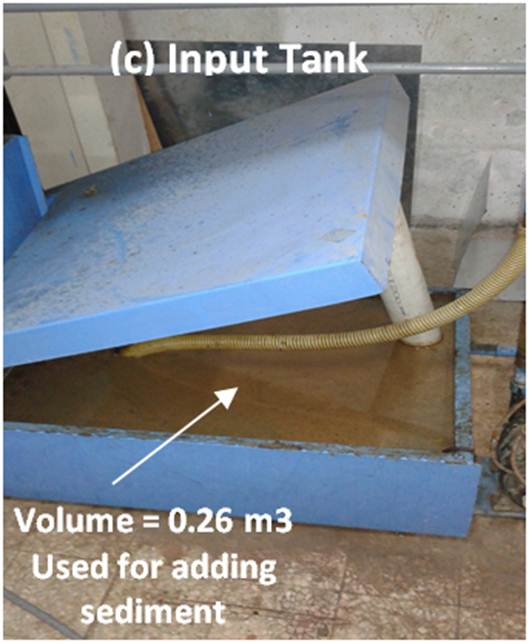Figure 4. Input tank with 0.26 m3.

First, three runs were exerted for measuring water velocity distribution without any sediments (pure water) using ADV instrument at water depths of 11 cm (h/D = 0.55), 13 cm (h/D = 0.65) and 15 cm (h/D = 0.75) with a discharge of 1.5 lit. /sec. as a maximum discharge could be obtained in the used flume.

In which, h = water depth measured at the centerline of channel and D = diameter of used channel, equals 20 cm. After that, three different sediment concentrations, represents the values of total load, of 1000 gm, 1500 gm and 2000 gm were prepared from fine clay soil with average geometric mean size of d50=0.097 mm. Then, for each concentration, three runs were exerted for the selected thee water depths.

Through the run time, the quantity of bed load was estimated with time propagation by weight (gm) at the trap used at the end of channel. The rate of increasing bed load with run time is the same rate of increasing suspended load. The run time was estimated to be 3.0 "three" hours.

After three hours from starting run, the concentration of suspended load remains constant and then, the "ADV" instrument, figure 5 and figure 6, with accuracy equals 1% of measured velocity and resolution of 0.01 cm/sec., was used for measuring water velocity distribution.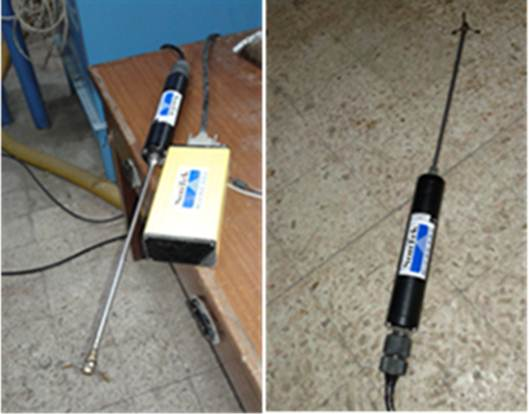Figure 5. Acoustic Doppler Velocimeter.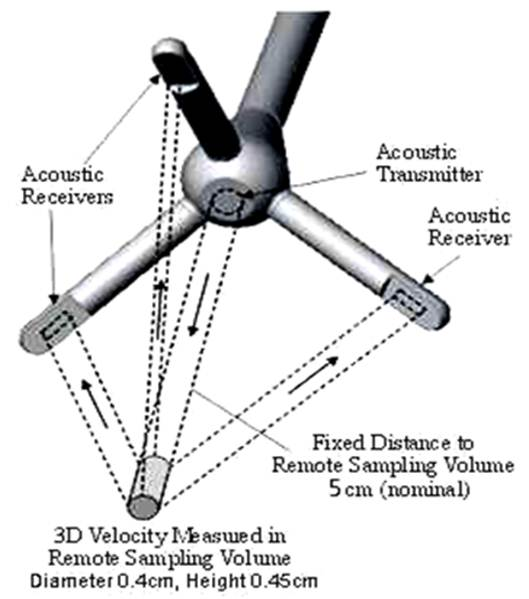Figure 6. Sketch of measuring a sample.

Acoustic Doppler Velocimeter Instrument

Sensor mounted on a 25cm stem.

High sampling rates: up to 50 Hz.

Small sampling volume: less than 0.1 cm3

Accuracy: 1% of measured range

Range of velocity: 1 mm/s to 2.5 m/s

Low-flow performance: excellent

No recalibration needed.

3. Sedimentation Rates with Time Propagation

To explain the change of suspended sediment concentration in water with time, the sediment particles was collected in the trap zone at the end of the channel after 0.5 hr., 1.0 hr., 2 hrs. and 3 hrs. from starting run. Then, the quantity of suspended load that moves with water equals the difference between total load added and the collected quantity of bed load. Figures 7 through 9 explain the variation of bed and suspended load with time at different total load concentrations.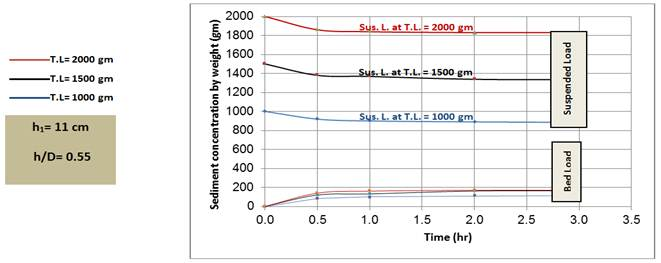Figure 7. Bed and suspended load variation with time at water depth-diameter ratio h/D =0.55.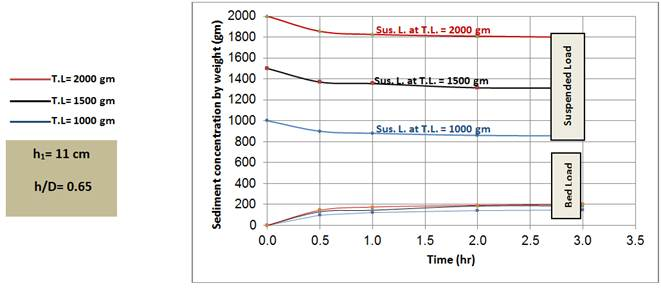Figure 8. Bed and suspended load variation with time at water depth-diameter ratio h/D =0.65.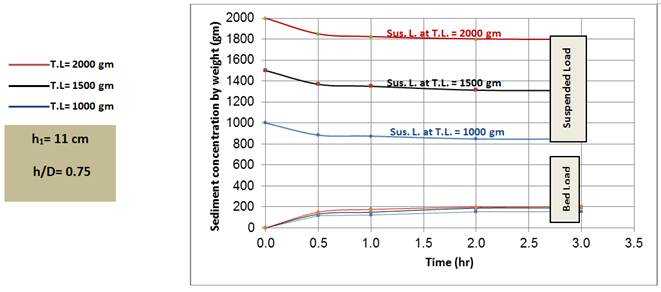Figure 9. Bed and suspended load variation with time at water depth-diameter ratio h/D =0.75.

The rate of increasing bed load with time for different total loads concentrations at the three used water depth-diameter ratios was explained through the following third degree polynomial equations.

·     Total load = 1000 gm

q (gm) = 18.81 t3 – 109.41 t2 + 197.41 t

R2= 0.99, ath/D =0.55                          (1)

q (gm) = 21.29 t3 – 126.11 t2 + 235.33 t

R2= 0.98at h/D =0.65                            (2)

q (gm) = 23.32 t3 – 138.75 t2 + 257.38 t

R2= 0.96, at h/D =0.75                           (3)

·     Total load = 1500 gm

q (gm) = 24.23 t3 – 143.88 t2 + 269 t

R2= 0.96, at h/D =0.55                        (4)

q (gm) = 23.92 t3 – 146.47 t2 + 286 t

R2= 0.96, at h/D =0.65                        (5)

q (gm) = 25.02 t3 – 151.95 t2 + 295 t

R2= 0.97, h/D =0.75                       (6)

·     Total load = 2000 gm

q (gm) = 33.96 t3 – 195.3 t2 + 337.31 t

R2= 0.97, at h/D =0.55                      (7)

q (gm) = 34.25 t3 – 197.4 t2 + 351.54 t

R2= 0.99, at h/D =0.65                      (8)

q (gm) = 33.80 t3 – 197.3 t2 + 355.67 t

R2= 0.97, at h/D =0.75                       (9)

In which, q = bed load (gm) and t= time by hour.

The aforementioned equations could be applied only at the discharge of 1.5 lit./sec. and for circular pipe channel, at the centerline, with h/D ratios ranged from 0.55 to 0.75.

The final quantities of bed load and the corresponding suspended load sediments after three hours from starting operating were concluded in the following table.

Table (1) presents the final values of bed load and the corresponding suspended load at different total loads.

Table 1. Final values of bed load and the corresponding bed load at different total loads.

 T.L.=1000 (gm) T.L.=1500 (gm) T.L.=2000 (gm) h/D h (cm) B.L. (gm) * Sus. L. (kg/m3) B.L. (gm) Sus. L. (kg/m3) B.L. (gm) Sus. L. (kg/m3) 0.55 11 115 3.40 165 5.13 170 7.04 0.65 13 145 3.28 186 5.05 202 6.92 0.75 15 152 3.26 190 5.03 203 6.91

Suspended load concentration was calculated in the aforementioned table as:-

- Example:

-If the used total load equals 1000 gm, bed load trapped at the final run time is 115 gm and the dimensions of input tank equal 67.5 cm × 154 cm × 25 cm (0.26 m3). Then, Suspended load concentration = (1000 – 115) / (0.26×1000) = 3.40 kg/m3.

4. Velocity Distribution

Vertical velocity distribution at the final obtained different suspended load concentrations given in table (1) for the three selected water depth-diameter ratio h/D = 0.55, 0.65 and 0.75 were presented in figures 10 through 12.

In these figures the concentration of suspended load sediments affects obviously the water velocity.

Then, three final equations were deduced to associate between the concentration of suspended load by (kg/m3) and the mean water velocity measured by ADV instrument by (cm/sec.).

These equations are polynomial functions from the second degree and must be applied only for circular channel and discharge of 1.5 lit. /sec. at the centerline.

Vm (cm/sec.) = 0.0167 C2 - 0.2702 C + 8.6273

R² = 0.9993at [h/D = 0.55]                     (10)

Vm (cm/sec.) = 0.0211C2 - 0.3257C + 9.0092

R² = 0.9993at [h/D = 0.65]                    (11)

Vm(cm/sec.) = 0.0564 C2 - 0.7303 C + 8.708

R² = 0.996at [h/D = 0.75]                       (12)

In which,

V m = mean velocity at the section by cm/sec., and

C = concentration of suspended load by kg/m3.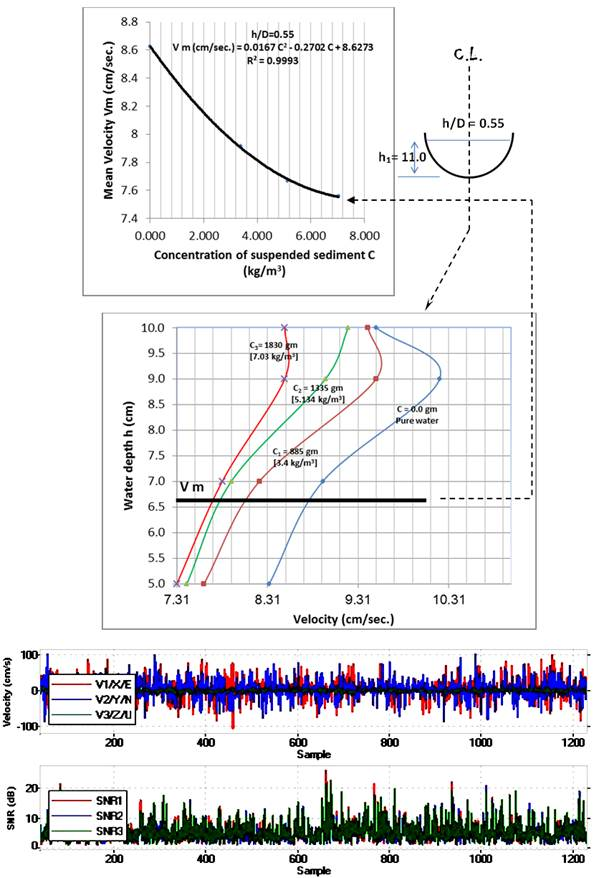Figure 10. Velocity distribution at for final suspended load concentrations at [h/D = 0.55].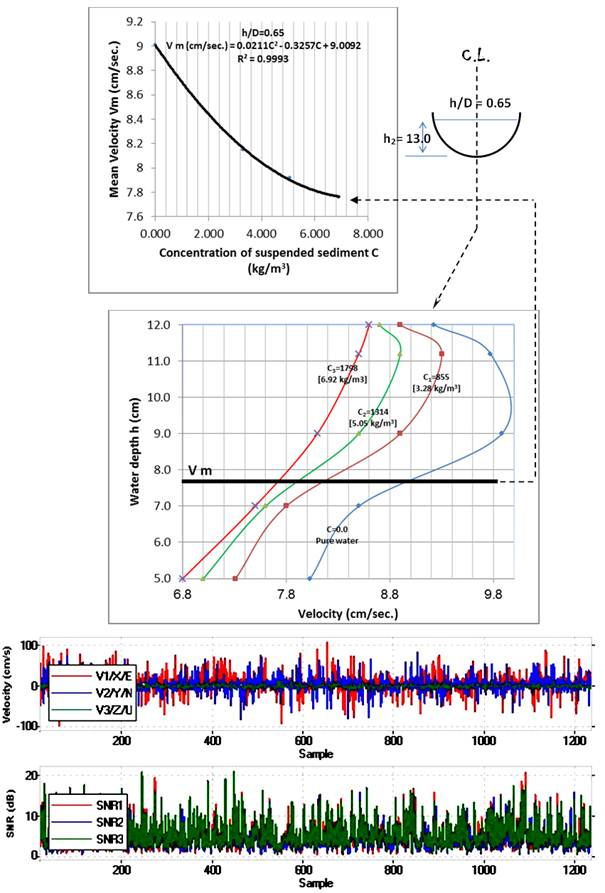Figure 11. Velocity distribution at for final suspended load concentrations at [h/D = 0.65].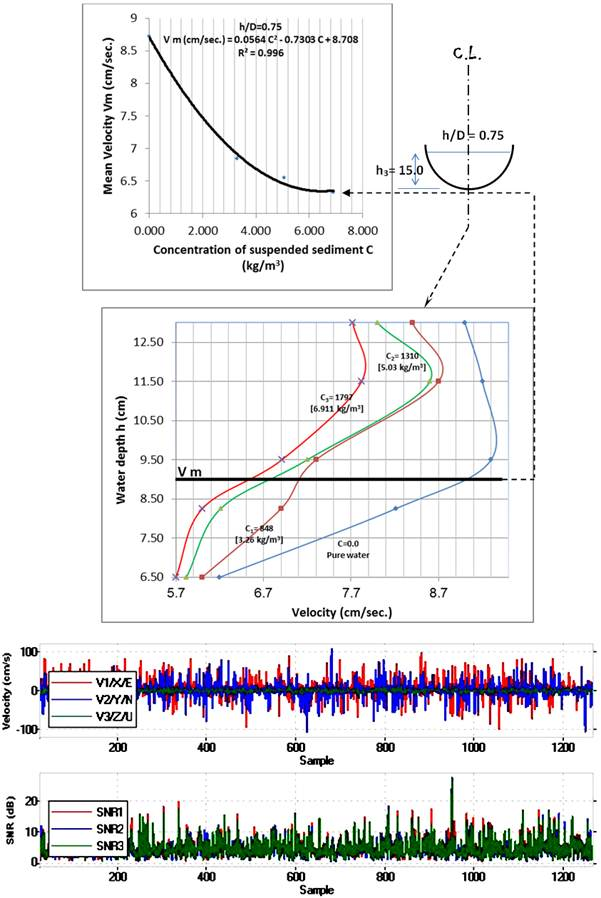Figure 12. Velocity distribution at for final suspended load concentrations at [h/D = 0.75].

5. Sediment with Water Depth

Figure 13 shows the relationship between water depth and final bed and suspended loads.

From this figure, it was appeared that with the increasing of water depth, the bed load increases and consequently decreases in suspended load concentration.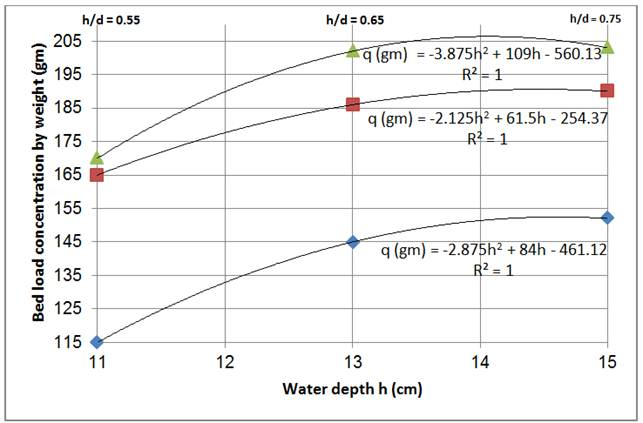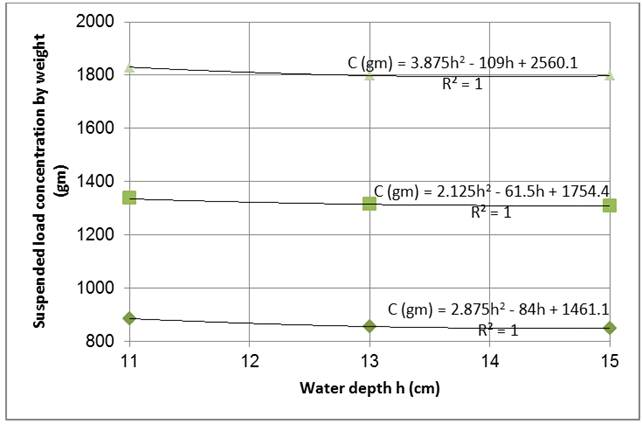Figure 13. Relationship between bed and suspended load and water depth.

Some of velocity results for the three selected water depth-diameter ratio (0.55, 0.65 and 0.75) were given in the form of following tables.

Table 2. Velocity values at h/D =0.55.

 h1=11 cm Run 1 Run 2 Run 3 Run 4 Sus. L. C=0.0 885 1335 1830 h (cm) V (cm /sec.) V (cm /sec.) V (cm /sec.) V (cm /sec.) 5.0 8.33 7.61 7.42 7.31 7.0 8.92 8.22 7.91 7.81 9.0 10.21 9.51 8.95 8.5 10.0 9.51 9.42 9.2 8.5 Vm 8.625 7.915 7.665 7.56

Table 3. Velocity values at h/D =0.65.

 h1=13 cm Run 5 Run 6 Run 7 Run 8 Sus. L. C=0.0 855 1314 1798 h (cm) V (cm /sec.) V (cm /sec.) V (cm /sec.) V (cm /sec.) 5.0 8.03 7.31 7 6.8 7.0 8.5 7.8 7.6 7.5 9.0 9.88 8.9 8.5 8.1 11.2 9.77 9.3 8.9 8.5 12.0 9.22 8.9 8.7 8.6 Vm 9.012 8.151 7.921 7.758

Table 4. Velocity values at h/D =0.75.

 h1=15 cm Run 9 Run 10 Run 11 Run 12 Sus. L. C=0.0 848 1310 1797 h (cm) V (cm /sec.) V (cm /sec.) V (cm /sec.) V (cm /sec.) 6.50 6.2 6 5.82 5.7 8.25 8.21 6.9 6.21 6 9.50 9.3 7.3 7.2 6.91 11.50 9.2 8.7 8.6 7.82 13.00 9 8.4 8 7.72 Vm 8.721 6.845 6.547 6.321

6. Conclusion

This experimental work was carried out to study the effect of different concentrations of fine sediments on the velocity distribution in circular cross section.

An ADV instrument was used for measuring the vertical velocity distribution at the centerline of channel.

Three values of total loads of 1000 gm, 1500 gm and 2000 gm were added to the input upstream tank of the used channel with 0.26 m3 volume. They all fine sediments.

A trap was designed at the end of the channel in order to find separately the final concentrations of suspended load and bed load.

Three water depths were used 11 cm, 13 cm and 15 cm to give depth-diameter ratios of 0.55, 0.65 and 0.75.

The final deduced time of each run that used for separating between bed and suspended loads was three hours.

It was concluded that, with the increasing of suspended load, the water velocity decreases obviously because the values of fall velocity will increase and consequently cause the increase in turbulent shear stress.

The increasing of water depth with the same discharge causes the increase of bed load and consequently the decrease in the corresponding suspended load concentrations.

Three final equations were deduced to associate between the concentration of suspended load by (kg/m3) and the mean water velocity measured by ADV instrument by (cm/sec.) for circular cross section as:

Vm (cm/sec.) = 0.0167 C2 - 0.2702 C + 8.6273

R² = 0.9993at [h/D = 0.55]

Vm (cm/sec.) = 0.0211C2 - 0.3257C + 9.0092

R² = 0.9993at [h/D = 0.65]

Vm (cm/sec.) = 0.0564 C2 - 0.7303 C + 8.708

R² = 0.996at [h/D = 0.75]

References

1. Al-Khafif S. "Study of open channels degradation and corresponding bed roughness", Ph.D. thesis, University of California, 1965.
2. Coles D. "The law of wake in the turbulent boundary layer", Journal of Fluid Mech., Vol.1, pp. 191–226, 1956.
3. Coleman N. "Velocity profiles with suspended sediment", Journal of Hydraulics Research, Vol. 19 (3), pp. 211–229, 1981.
4. Einstein H. and Chien N. "Effects of heavy sediment concentration near the bed on the velocity and sediment distribution", Report. No. 8, University of California, Berkeley, CA, 1955.
5. Grant, J., Walker, T. R., Hill P. S., Lintern, D. G. "BEAST-A portable device for quantification of erosion in intact sediment cores", Methods in Oceanography. DOI: 10.1016/j.mio.2013.03.001, 2013.
6. Itakura T. and Kishi T. "Open channel flow with suspended sediments", Journal of Hydraulics Division, ASCE, Vol. 106 (8), pp. 1325–1343, 1980.
7. Lavelle J. and Thacker W. "Effects of hindered settling on sediment concentration profiles", Journal of Hydraulics Research, IAHR, Vol. 16 (4), pp. 347–355, 1978.
8. Mazumder B. and Ghoshal K. "Velocity and concentration profiles in uniform sediment-laden flow", Applied Mathematical Modeling, Vol. 30 (2), pp. 164–176, 2006.
9. Milad K., Kyle S. and Qin Q. "A Literature Review on the Sediment Transport Process in Shallow-Grade Culverts and Storm Sewers", Proceedings of the ASEE Gulf-Southwest Annual Conference Organized by The University of Texas at San Antonio, 2015.
10. Newton C. "An experimental investigation of bed degradation in an open channel", Transcript, Boston Society of Civil Engineers, pp.28-60, 1951.
11. Nezu I. and Rodi W. "Open-channel flow measurements with a Laser Doppler Anemometer", Journal of Hydraulics Engineering, ASCE, Vol. 112, pp. 335–355, 1986.
12. Nezu I. and Nakagawa H. "Turbulence in open-channel flows", IAHR Monograph A. A. Balkema Publishers, Rotterdam, 1993.
13. Pellachini I. "Modeling fine sediment transport over an immobile gravel bed", Trento: Unitn - eprints. 2011.
14. Schlichting H. and Gersten K. "Boundary layer theory", (8th ed.) Springer, 2000.
15. Suryanarayana B. and Shen W. "Laboratory study of degradation and aggradation", Journal of Waterways, Harbors and Coastal Engineering Division, Vol.97, pp.615-630, 1971.
16. Woo H., Julien P. and Richardson E. "Suspension of large concentration of sands", Journal of Hydraulics Engineering, ASCE, Vol. 114, pp. 888–898, 1988.
17. Vanoni V. "Transportation of suspended sediment by water", Trans. ASCE, Vol. 111, pp. 67–133, 1946.

 Contents 1. 2. 3. 4. 5. 6.
Article ToolsAbstractPDF(1162K)